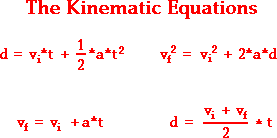# Determine the Acceleration

smashbrohamme
The ball of a pinball machine is accelerated from rest to 11in/s in a distance of 4in. Determine the acceleration of the ball

V=Vo +at
A= Change of Velocity/ Change of Time

The answer is 15.1 in/s^2

The time the pinball traveled was 4inches/11in - .36sec
I cant seem to get what I am doing wrong but I am not getting 15.1in/s^2
I am doing 11in/s= 0+a(.36) A= 30.5
I also tried doing change of velocity/change of time which is just 11/.36 as well.

Since Vo= 0 The change of velocity is simply 11/in/s^2 and the change of time is simply
.36seconds

## Answers and Replies

Homework Helper
Gold Member
The ball of a pinball machine is accelerated from rest to 11in/s in a distance of 4in. Determine the acceleration of the ball

V=Vo +at
A= Change of Velocity/ Change of Time

The answer is 15.1 in/s^2

The time the pinball traveled was 4inches/11in - .36sec
I cant seem to get what I am doing wrong but I am not getting 15.1in/s^2
I am doing 11in/s= 0+a(.36) A= 30.5
I also tried doing change of velocity/change of time which is just 11/.36 as well.

Since Vo= 0 The change of velocity is simply 11/in/s^2 and the change of time is simply
.36seconds
You are not calculating the time correctly. The ball is not travelling at a constant speed of 11 in/sec...its speed varies from 0 to 11 in/sec.. You can calculate its average speed to find the time, or use one of the other kinematic equations.

Lavid2002
This is how I was taught. Write out everything you know first. (Dont skip steps)
Vo, initial velocity
Vf, final velocity
∆x, delta x, or displacement x

We need to find
a, acceleration

Now we take a look at the four of our kinematics,In order to solve for a variable in an equation we must have all the variables but one.

We have all the variables for this equation
Vf^2=Vo^2+2a∆x

Except acceleration, but thats what were solving for : )

P.S. Our initial velocity is 0 So we can simplify this equation
Vf^2=2a∆x

Last edited:
Homework Helper
Gold Member
This is how I was taught. Write out everything you know first. (Dont skip steps)
Vo, initial velocity
Vf, final velocity
∆x, delta x, or displacement x

We need to find
a, acceleration

P.S. Our initial velocity is 0 So we can simplify this equation
Vf^2=2a∆x
Correct! Solve for a.